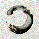TradingView
3444 visningar
Here simply calculate the CCI of the volume , this will use to measure the strength of the trend of the volume

Background:
I think the volume as the price could be represented by candles or other graphic to use indicators and strengthen their analysis, due to lack of registration of this it is first necessary to calculate a volume graph, if the candle traditionally negative price brand then the total volume is taken as negative for the period. An example of this is in the On Balance Volume indicator, the problem is that there is no way to analyze the volume using other methods. An approximate volume of the spread could be the use of the price spread to make a synthetic behavior

As traditionally is observed if Open> Close then the candle and the volume will be negative and vice versa; the next step, is estimate the amounts of the candle necessary to calculate the ratio to use for the volume and thus idealize their spread within the candle:

VLOW = Volume x Low
vHigh = x High Volume
VOpen = vClose
vClose = Volume x Close

This graph can show a stable synthetic form of fluctuations in the volume trend affected by price.
ideas, comments and suggestions (or corrections).They are always welcome
Open-source script

In true TradingView spirit, the author of this script has published it open-source, so traders can understand and verify it. Cheers to the author! You may use it for free, but reuse of this code in a publication is governed by House Rules. You can favorite it to use it on a chart.

Want to use this script on a chart?
```//@version=2
study("CCI de Volumen")
/// INPUTS
shortlen = input(28, title="signal",minval=1)
longlen = input(56, title="Profundidad",minval=1)
/// Values
volumex=volume//*(close>close ? 1 : -1)
V_OPEN  = V_CLOSE
V_CLOSE = close*volumex
V_HIGH  = max(max(high*volumex,V_CLOSE),V_OPEN)
V_LOW   = min(min(low*volumex,V_OPEN),V_CLOSE)
V_HL2   = ( V_HIGH + V_LOW ) / 2

ccx=cci(V_CLOSE,longlen)
plot(ccx, color=blue)
plot(sma(ccx,shortlen),color=red, transp=30, linewidth=2)
hline(0, title="Axis", color=gray, linestyle=dashed,linewidth=2)
sc=hline(100, title="Sobre-Compra", color=silver, linestyle=dotted)
ei=hline(200, title="Exuberancia Irracional", color=silver, linestyle=solid)
sv=hline(-100, title="Sobre-Venta", color=silver, linestyle=dotted)
pp=hline(-200, title="Panico Psicotico", color=silver, linestyle=solid)
fill(ei,pp, color=silver)
fill(sc,sv, color=gray)```

## Kommentarer//@version=2
study("CCI of Volume")
/// INPUTS
shortlen = input(28, title="signal",minval=1)
longlen = input(56, title="Window",minval=1)
/// Values
volumex=volume//*(close>close ? 1 : -1)
V_OPEN = V_CLOSE
V_CLOSE = close*volumex
V_HIGH = max(max(high*volumex,V_CLOSE),V_OPEN)
V_LOW = min(min(low*volumex,V_OPEN),V_CLOSE)
V_HL2 = ( V_HIGH + V_LOW ) / 2

ccx=cci(V_CLOSE,longlen)
plot(ccx, color=blue)
plot(sma(ccx,shortlen),color=red, transp=30, linewidth=2)
hline(0, title="Axis", color=gray, linestyle=dashed,linewidth=2)
sc=hline(100, title="Over-Buy", color=silver, linestyle=dotted)
ei=hline(200, title="Ridiculous Exuberance", color=silver, linestyle=solid)
sv=hline(-100, title="Over Sell", color=silver, linestyle=dotted)
pp=hline(-200, title="Psychopathic panic", color=silver, linestyle=solid)
// the terms Ridiculous and Psychopathic are an non-formal reference to the animal spirits, to the merely psychological, irrational and exuberant sense of the economic forces narrated by Greenspan.
fill(ei,pp, color=silver)
fill(sc,sv, color=gray)
Svara
Profil Profilinställningar Konto och fakturering Hänvisade vänner Mynt Mina kölappar Hjälpcenter Privata meddelanden Chatt Logga ut